# Frank Solutions for Chapter 12 Practical Work Class 10 Chemistry ICSE

1. How will you identify ?
(a) Chloride ion
(b) Chlorine gas
(c) Sulphur dioxide gas
(d) A soluble carbonate
(e) An amphoteric hydroxide

(a) Chloride ion: A small amount of the salt is taken in a test tube and conc. H2SO4 is added to it and then test tube is warmed, if a colourless gas with pungent odour is evolved then chloride ions are present in the salt. It can be confirmed by bringing a glass rod dipped in ammonia solution near the gas evolved, if dense white fumes are formed then presence of chloride ions is confirmed.

(b) It is a greenish yellow coloured gas with sharp pungent smell. It turns moist blue litmus paper red and finally bleaches it.

(c) It is a colourless gas with a smell of burnt sulphur. It turns moist blue litmus paper red.

(d) A small quantity of salt is taken in a test tube, dilute sulphuric acid is added to it. If a brisk effervescence is observed, then the gas is passed through lime water, If carbonate ion is present in the salt, the solution turns milky.

(e) Zinc hydroxide is an amphoteric hydroxide. It can be identified by treating the salt solution with sodium hydroxide, if a white ppt. is formed and if it gets dissolved in excess of sodium hydroxide then it may be zinc hydroxide .

2. Name the following  :
(a) A green coloured carbonate.
(b) A steel grey coloured solid non-metal.
(c) A nitrate which has no water of crystallization.
(d) A sulphate insoluble in water.

(a) Copper carbonate
(b) Selenium
(c) Nitre: a nitrate of potassium
(d) Anhydrous calcium sulphate

3. Give two tests for each of the following :
(a) NH3
(b) Oxygen
(c) Water vapour

(a) NH3: It has a strong pungent smell and turns moist red litmus blue. It can also be tested by bringing a glass rod dipped in conc. HCI in contact with the gas, if the gas is ammonia then it will produce dense white fumes near the glass rod.

(b) Oxygen: It is an odourless and colourless gas and turns alkaline pyrogallol brown. It can also be tested by bringing a lighted splinter near the gas, if the splinter starts glowing, the gas is oxygen.

(c) Water Vapour: These are colourless vapours and are neutral to litmus. These can be tested by first condensing on the walls (cooler parts) of the test tube and then by adding anhydrous copper sulphate to the collected liquid , if the white colour of copper sulphate changes to blue, then the gas is water vapour.

4. Write the steps needed for flame test ?

Flame test :

1. Make a loop at the tip of the platinum wire and dip it in conc. HCl.
2. Put it on the non luminous part of the flame, to see if gives colour. Repeat the process till it gives no colour to the flame.
3. Prepare a paste of the given salt in a watch glass using conc. HCl.
4. Load the loop of the wire with this prepared paste and introduce it into the non luminous flame of the bunsen burner and then observe the colour of the flame indicating different elements.

5. What happens when
(a) NH3 solution is added to CuSO4 solution drop by drop and then in excess.
(b) Caustic soda solution is added to Cu(NO3)2 solution and product boiled.
(c) Common salt solution is added to silver nitrate solution and NH3 solution add to it.
(d) Lead nitrate solution treated with calcium chloride solution and products are heated and cooled.

(a) When NH3 solution is added to CuSO4 solution drop by drop, ammonium ions completely react with copper sulphate and precipitates of copper hydroxide are formed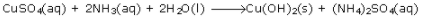But when we add excess of ammonia, the precipitate dissolves and a soluble complex is formed.(b) When Caustic soda solution is added to Cu(NO3)2 solution, the following reaction takes place :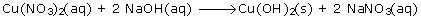when we heat it further, the greenish blue copper hydroxide decomposes form slightly black ppt. of copper oxide.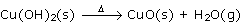(c) Common salt solution is added to silver nitrate solution.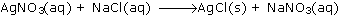Common salt in NaCl, in aqueous medium it ionizes to form Na+ and Cl- ions.6. Write sqauential observation for effect of heat on
(a) Copper nitrate
(c) Ammonium chloride

(a)

1. Bluish green crystalline solid, on heating, melts to form a bluish green mass and gives off steamy vapours which condense on the cooler part of the test tube.
2. On further heating, the bluish green mass changes to a black residue.
3. It gives off a reddish brown gas and gives a gas which rekindles a glowing splinter, i.e. oxygen.
(b)

1. A white solid turns yellow on heating.
2. Gives off a gas which extinguishes a burning wooden splinter.
3. Gas evolved turns lime water milky.
(c)

1. When ammonium chloride is heated in a test tube, the lighter ammonia gas will emerge first and turn a piece of moist red litmus paper blue.
2. Hydrogen chloride coming up next will change the litmus paper from blue back to red.

7. How will you distinguish between the two black samples, CuO and MnO2 with a chemical test ?

Add concentrated hydrochloric acid to both the samples. Only MnO2 releases greenish yellow chlorine gas.

8. A white solid a when heated with sodium hydroxide solution, gives a pungent gas B, which turns red litmus blue. The solid, when dissolved in dilute nitric acid and treated with silver nitrate gives a white precipitate of C which is soluble in an ammonia solution.

C is silver chloride which is soluble in ammonia.
Pungent smelling gas B is ammonia.
White solid A is ammonium chloride9.  Write the approximate colour of the following samples with the universal indicator :
(a) Distilled water
(b) Acid rain
(c) Soap solution
(d) Soil containing slaked lime
(e) Gastric juice

(a) Distilled water : same as that of universal indicator
(b) Acid rain : red
(c) Soap solution : purple
(d) soil containing slaked lime : green
(e) Gastric juices : orange

10. Using sodium hydroxide solution, how would you distinguish :
(a) Ammonium sulphate from sodium sulphate.
(b) Zinc nitrate solution from calcium nitrate solution .
(c) Iron (II) chloride from Iron (III) chloride.

11. Describe in each case, one chemical test that would enable you to distinguish between following pairs of chemicals. Describe what happens with each chemical or state 'no visible reaction'.
(i) Sodium chloride solution and sodium nitrate solution.
(ii) Sodium sulphate solution and sodium chloride solution.
(iii) Calcium nitrate solution and zinc nitrate solution.

(i) Sodium chloride solution and sodium nitrate solution can be distinguished by using conc. Sulphuric add. To the salt solution, add freshly prepared ferrous sulphate solution and pour a few drops of conc. H2SO4  along the sides of the tube. If it's sodium nitrate solution then a brown ring would appear at the junction of the two liquid layers. But if its sodium chloride solution, it would not undergo any visible reaction.

(ii) Sodium sulphate solution and sodium chloride solution can be distinguished by using barium chloride solution. Barium chloride solution on being added to sodium sulphate solution forms a white precipitate which is insoluble in conc. HCI. Whereas sodium chloride shows no reaction with barium chloride solution.

(iii) Calcium nitrate solution and zinc nitrate solution can be distinguished by sodium hydroxide. Sodium hydroxide reacts with zinc nitrate and zinc hydroxide is formed which is soluble in excess of sodium hydroxide.Sodium hydroxide reacts with calcium nitrate and forms white ppt. of calcium hydroxide insoluble in excess of sodium hydroxide.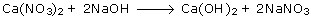12. State the effect of adding a small amount of : (a) sodium hydroxide (b) ammonium hydroxide followed by an excess in each case to samples of each of the salt solutions.
(i) Calcium nitrate [ small amount ________ in excess ______].
(ii) Zinc nitrate [small amount _______ in excess ______ ].
(iii) Lead nitrate [ small amount _______ in excess _______].

13. State what do you observe when ammonium hydroxide to Iron (III) sulphate solution.

14. From the formulae listed below, choose, one, corresponding to the salt having the given description :AgCl, CuCO3, CuSO4∙5H2O, KNO3, NaCl, NaHSO4, Pb(NO3)2, ZnCO3, ZnSO4∙ 7H2O.
On heating this, salt changes from green to black.

On heating CuCO3, CuO is formed which is black in colour.

15. (i) How would you distinguish between Zn2+ and Pb2+ using ammonium hydroxide solution ?
(ii) Copy and complete the following table which refers to the action of heat on some carbonates :

 Carbonate Colour of residue on cooling Zinc carbonateLead carbonateCopper carbonate

16. Write the observation for the following:
(i) NaOH is added drop-wise till in excess to a solution of zinc sulphate.
(ii) NH4OH is added first in a small quantity and then in excess to a solution of copper sulphate.
(iii) Excess NH4OH is added to a substance obtained by adding hydrochloric add in silver nitrate solution.
(iv) Moist starch iodide paper is put on the mouth of a test tube containing chlorine gas.
(v) A paper dipped in potassium permanganate solution is put on the mouth of a test tube containing sulphur dioxide gas.
(vi) Decomposition of bicarbonates by dil. H2SO4  .(iv) Chlorine gas turns moist starch iodide paper black.
(v) Purple colour of potassium permanganate gets discharged.
(vi) Brick effervescence is observed and colourless and odourless CO2 gas is evolved in both the cases.

17. Sodium hydroxide solution is added first in a small quantity, then in excess to the aqueous salt solutions of copper (II) sulphate, zinc nitrate, lead nitrate , calcium chloride and iron (III) sulphate. Copy the following table and write the colour of the precipitate in (i) to (v) and the nature of the precipitate (soluble or insoluble ) in (vi) to (x).

 Aqueous salt solution Colour of precipitate when NaOH is added in a small quantity Nature of precipitate (soluble or insoluble )when NaOH is added in excess Copper (II) sulphateZinc nitrateLead nitrateCalcium chlorideIron (III) Sulphate (i)(ii)(iii)(iv)(v) (vi)(vii)(viii)(ix)(x)

 Aqueous salt solution Colour of precipitate when NaOH is added in a small quantity Nature of precipitate (soluble or insoluble) when NaOH is added in excess Copper (II) sulphateZinc nitrateLead nitrateCalcium chlorideIron (III) Sulphate (i) Pale blue (ii) White(iii) White(iv) White curdy ppt.(v) Reddish brown (vi) insoluble (vii) soluble (viii) soluble(ix) insoluble (x) insoluble

18. A solution of hydrogen chloride in water is prepared. The following substances are added to separate portions of the solution :

 S. No. Substance added Gas evolved Odour 1. Calcium carbonate2. Magnesium ribbon3. Manganese (IV) oxide with heating4. Sodium sulphide ____________ ________

 S. No. Substance added Gas evolved Odour 1. Calcium carbonate2. Magnesium ribbon3. Manganese (IV) oxide with heating4. Sodium sulphide Co2  H2  Cl2  H2S odourlessodourlesspungentsmell of rotten eggs

19. The questions (i) to (v) refer to the following salt solutions listed (a) to (f):
(a) Copper nitrate
(b) Iron (II) sulphate
(c) Iron (III) chloride
(e) Magnesium sulphate
(f) Zinc chloride
(i) Which two solutions will give a white precipitate when treated with dilute hydrochloric acid followed by barium chloride solution?
(ii) Which two solutions will give a white precipitate when treated with dilute nitric acid followed by silver nitrate solution?
(iii) Which solution will give a white precipitate when either dilute hydrochloric acid or dilute sulphuric acid is added to it?
(iv) Which solution becomes a deep/inky blue colour when excess of ammonium hydroxide is added to it?
(v) Which solution gives a white precipitate with excess ammonium hydroxide solution?

(i) Magnesium sulphate, Iron(II) sulphate
(ii) Zinc chloride, Iron (III) chloride
(iv) Copper nitrate
(v) Zinc chloride

20. (a) State the colour of the residue formed when nitrates of
1. Calcium
2. Zinc
4. Copper are strongly heat
(b) Give one test each to distinguish between the following pairs of chemicals.
1. Zinc nitrate solution and calcium nitrate solution.
2. Sodium nitrate solution and sodium chloride solution.
3. Iron (III) chloride solution and copper chloride solution.

(a) 1. Calcium: white
2. Zinc: white
4. Copper: black

(b) 1. Zinc nitrate and calcium nitrate solution can be distinguished by reaction with ammonium hydroxide. Zinc forms a white gelatinous ppt. whereas there is no precipitation of calcium hydroxide even with excess of ammonium hydroxide.1. (a) Sodium chloride solution and sodium nitrate solution can be distinguished by using conc. Sulphuric acid. To the salt solution, add freshly prepared ferrous sulphate solution and pour a few drops of conc. H2SO4 along the sides of the tube. If it's sodium nitrate solution then a brown ring would appear at the junction of the two liquid layers. But if its sodium chloride solution, it would not undergo any visible reaction.
2. Iron (III) chloride solution and copper chloride solution can be distinguished by using ammonium hydroxide. Copper forms a blue ppt. of Cu(OH)2 which is soluble in excess of ammonium hydroxide.
CuCl2  + 2NH4OH ⟶ Cu(OH)2 + 2NH4Cl
Whereas iron (III) forms a reddish brown ppt. of Fe(OH)3 which is insoluble even in excess of ammonium hydroxide.
FeC13  + 3NH4OH ⟶ Fe(OH)3  + 3NH4Cl

21.

 Column A Column B 1. A substance that turns moist starch iodide paper blue. C. Chlorine 2. A compound which releases a reddish brown gas on reaction with concentrated sulphuric acid and copper turnings. D. Copper nitrate 3. A solution of this compound gives a dirty green precipitate with sodium hydroxide. E. Ferrous sulphate 4. A compound which on heating with sodium hydroxide produces a gas which forms dense white fumes with hydrogen chloride. A. Ammonium sulphate 5. A white solid which gives a yellow residue on heating B. Lead carbonate

Match the following :

 Column A Column B 1. A substance that turns moist starch iodide paper blue. A. Ammonium sulphate 2. A compound which releases a reddish brown gas on reaction with concentrated sulphuric acid and copper turnings. B. Lead carbonate 3. A solution of this compound gives a dirty green precipitate with sodium hydroxide. C. Chlorine 4. A compound which on heating with sodium hydroxide produces a gas which forms dense white fumes with hydrogen chloride. D. Copper nitrate 5. A white solid which gives a yellow residue on heating E. Ferrous sulphate

22. Salts A, B, C, D and E undergo reactions (i) to (v) respectively. Identify the anion present in these salts on the basis of these reactions. Tabulate your answers in the format given below:
(i) When silver nitrate solution is added to a solution of A, a white precipitate, insoluble in dilute nitric acid, is formed.
(ii) Addition of dilute hydrochloric acid to B produces a gas which turns lead acetate paper black.
(iii) When a freshly prepared solution of ferrous sulphate is added to a solution of C and concentrated sulphuric acid is gently poured from the side of the test, a brown ring is formed.
(iv) When dilute sulphuric acid is added to D, a gas is produced which turns acidified potassium dichromate solution from orange to green.
(v) Addition of dilute hydrochloric acid to E produces an effervescence. The gas produced turns limewater milky but does not affect acidified potassium dichromate solution.

 Salt Anion A B C D E

23. Choose the correct answer :

The salt which in solution gives a pale precipitate with sodium hydroxide solution and a white precipitate with barium chloride solution is :
A. Iron (III) sulphate
B. Iron (II ) sulphate
C. Iron (II) chloride
D. Iron (III) chloride

Iron (II) sulphate

24. Select from the list given (a to e) one substances in each case which matches the description given in parts (i) to (v). (Note : Each substance is used only one in the answer)
(a) Nitroso Iron (II)
(b) Iron (III) chloride
(c) Chromium sulphate
(e) Sodium chloride
(i) A compound which is deliquescent
(ii) A compound which is insoluble in cold water, but soluble in hot water
(iii) The compound responsible for the brown ring during the brown ring test of nitrate iron
(iv) A compound whose aqueous solution is neutral in nature
(v) The compound which is responsible for the green colouration when sulphur dioxide is passed through acidified potassium dichromate solution

(i) Iron (III) chloride
(iii) Nitroso iron (II) sulphate
(iv)Sodium chloride
(v) Chromium sulphate

25. What would you observe in the following cases:
Ammonium hydroxide is first added in a small quantity and then in excess to a solution of copper sulphate.

On addition of ammonium hydroxide in a small quantity, a blue-coloured copper hydroxide precipitate is formed. This copper hydroxide of light blue colour dissolves in excess of ammonium hydroxide to yield a deep blue solution.

26. Sodium hydroxide solution is added to the solutions containing the ions mentioned in List X. List Y gives the details of the precipitate. Match the ions with their coloured precipitates.

 List X List Y (i) Pb2+(ii) Fe2+(iii) Zn2+(iv) Fe3+(v) Cu2+(vi) Ca2+ (A) Reddish Brown(B) White insoluble in excess(C) Dirty green(D) White soluble in excess(E) White soluble in excess(F) Blue

(i) D
(ii) C
(iii) E
(iv) A
(v) F
(vi) B

27.  State two observations when
(i) Lead nitrate crystals are heated in a hard glass test tube.
(ii) A few crystals of KNO3 are heated in a hard glass tube

(i) Lead nitrate decrepitates on heating; a yellow solid is formed and it fuses with glass. Lead nitrate decomposes to lead oxide, nitrogen dioxide and oxygen.
(ii) Oxygen is evolved.
2KNO3→ 2KNO2 + O2

28. Give a chemical test to distinguish between the following pairs of compounds:
(i) Sodium chloride solution and sodium nitrate solution
(ii) Hydrogen chloride gas and hydrogen sulphide gas
(iii) Calcium nitrate gas and sulphur diaoxide gas
(iv) Carbon dioxide gas and sulphur dioxide gas

(i) Add silver nitrate solution to both solutions. Sodium chloride will form a curdy white ppt., whereas sodium nitrate will not undergo any reaction.
(ii) Hydrogen chloride gas gives thick white fumes of ammonium chloride when a glass rod dipped in ammonia solution is held near the vapour of the acid, whereas no white fumes are observed in case of hydrogen sulphide gas.
(iii) Calcium nitrate forms no ppt. even with addition of excess of NH4OH, whereas zinc nitrate forms a white gelatinous ppt. which dissolves in excess of NH4OH.
(iv) Carbon dioxide gas has no effect on acidified KMnO4 or K2Cr2O7, but sulphur dioxide turns potassium permanganate from pink to colourless.

29. Distinguish between the following pairs of compounds using the test given with brackets :
Dilute sulphuric acid and dilute hydrochloric acid (using barium chloride solution)

Sulphuric acid precipitates the insoluble sulphate of barium from the solution of barium chloride.
BaCl2 + H2SO4→ BaSO4 + 2HCl
Dilute HCl does not react with barium chloride solution, and thus, no precipitate is produced in the reaction.

30. State the inference drawn from the following observations :
(i) On Carrying out the flame test with a salt P a brick red flame was obtained. What is the cation in P?
(ii) A gas Q turns moist lead acetate paper silvery black. Identify the gas Q.
(iii) pH of liquid R is 10. What kind of substance is R?
(iv) Salt S is prepared by reacting dilute sulphuric acid with copper oxide Identify S.

(i) On carrying out the flame test with a salt P, a brick red flame is obtained. Hence, the cation P is Ca2+.
(ii) A gas Q turns moist lead acetate paper silvery black. Hence, the gas is H2S.
(iii) pH of liquid R is 10. Hence, substance R is a base.
(iv) Salt S is prepared by reacting dilute sulphuric acid with copper oxide. Hence, salt S is copper sulphate.

31. State your observation in each of the following cases:
(i) When dilute hydrochloric acid is added to sodium carbonate crystals
(ii) When excess sodium hydroxide is added to calcium nitrate solution
(iii) At the cathode when acidified aqueous copper sulphate solution is electrolyzed with copper electrodes
(iv) When calcium hydroxide is heated with ammonium chloride crystals
(v) When moist starch iodide paper is introduced into chlorine gas

(i) Sodium carbonate crystals on reaction with dilute HCl form sodium chloride, water and carbon dioxide, which is evolved with brisk effervescence. This is a neutralisation reaction as sodium carbonate is a basic salt, while hydrochloric acid is an acid. The chemical equation for this reaction is as follows:
Na2CO3 + 2HCl → 2NaCl +H2O + CO2
(ii) Calcium nitrate solution on reaction with excess of sodium hydroxide produces calcium hydroxide and sodium nitrate. Calcium nitrate reacts with excess of sodium hydroxide to form a white precipitate of calcium hydroxide, which is sparingly soluble, and colourless sodium nitrate. The reaction is as follows:
Ca(NO3)2 + 2NaOH → Ca(OH)2 + 2NaNO3
(iii) Acidified aqueous copper sulphate solution is electrolysed with copper electrodes by electrolysis. The electrolysis of an aqueous solution of copper sulphate using copper electrodes (i.e. using active electrodes) results in the transfer of copper metal from the anode to the cathode during electrolysis. Copper sulphate is ionised in aqueous solution.
Chemical equation:
CuSO4 → Cu2+ + SO42-
The positively charged copper ions migrate to the cathode, where each gains two electrons to become copper atoms which are deposited on the cathode.
Cu2+ + 2e- → Cu
Hence, the colour of copper sulphate changes from blue to colourless.
(iv) When ammonium chloride is heated with calcium hydroxide, ammonia gas is released.
2NH4Cl + Ca(OH)2→ CaCl2 + 2NH3 + 2H2O
The liberated gas turns red litmus blue.
(v) When moist starch iodide paper is introduced into chlorine gas, chlorine oxidises iodide to iodine, which shows up as blue when it forms a complex with starch.

32. The following table shows the tests a student performed on four different aqueous solutions which are X,Y,Z and W. Based on the observations provided, Identify the cation present

 Chemical Test Observation Conclusion To Solution X, ammonium hydroxide is added in minium quantity first and then in excess A dirty white precipitate is formed which dissolves in excess to form a clear solution (i) To Solution Y, ammonium hydroxide is added in minimum quantity first and then in excess A pale blue precipitate is formed which dissolves in excess to form a clear inky blue solution (ii) To solution W, A small quantity of sodium hydroxide solution is added and then in excess A white precipitate is formed which remains insoluble (iii) To a salt Z, calcium hydroxide solution is added and then heated A pungent smelling gas turning moist red litmus paper blue is obtained (iv)

(i) Zn2+
(ii) Cu2+
(iii) Ca2+
(iv) NH4+

33. Identify the anion present in each of the following compounds :
(i) A salt M on treatment with concentrated sulphuric acid produces a gas which fumes in moist air and gives dense fumes with ammonia
(ii) A salt D on treatment with dilute sulphuric acid produces a gas which turns lime water milky but has no effect on acidified potassium dichromate solution
(iii) When barium chloride solution is added to salt solution E a white precipitate insoluble in dilute hydrochloric acid is obtained

(i) Chloride ion (Cl-)
(ii) Carbonate (CO32-)
(iii) Sulphate (SO42-

34. From the list of the following salts choose the salt that most appropriately fits the description given in the following :
[AgCl,MgCl2,NaHSO4,PbCO3,ZnCO3,KNO3,Ca(NO3)2]
(i) A deliquescent salt
(ii) An insoluble chloride
(iii) On heating this salt gives a yellow residue when hot and white when cold
(iv) On heating this salt, a brown coloured to prepare the following salts:

(i) A deliquescent salt: MgCl2
(ii) An insoluble chloride: AgCl
(iii) On heating, this salt gives a yellow residue when hot and a white residue when cold: ZnCO3
(iv) On heating this salt, a brown coloured gas is evolved: Ca(NO3)2

35. Give balanced chemical equations to prepare the following salts:
(ii) Sodium sulphate using dilute sulphuric acid
(iii) Copper chloride using copper carbonate

36. Identify the cations in each of the following case:
(i) NaOH solution when added to solution (A) gives a reddish brown precipitate
(ii) NH4OH solution when added to solution (B) gives white ppt. which does not dissolve in excess.
(iii) NaOH solution when added to solution (C) gives white ppt. which insoluble in excess37. Identify the gas evolved and give the chemical test in each of the following cases:
(i) Dilute hydrochloric acid reacts with sodium sulphite
(ii) Dilute hydrochloric acid reacts with iron (II) sulphide

(i) Sulphur dioxide
Freshly prepared K2Cr2O7 paper changes from orange to green.
(ii) Hydrogen sulphide
The gas released has a rotten egg smell.

38. Identify the salts P and Q from the observations given below:
(i) On performing the flame test salt P produces a lilac coloured flame and its solution gives a white precipitate with silver nitrate solution. Which is soluble in ammonium hydroxide solution.
(ii) When dilute HCl is added to a salt Q, a brisk effervescence is produced and the gas turns lime water milky. When NH4OH soltion is added to the above
mixture (after adding dilute HCl), it produces a white precipitate which is
soluble in excess NH4OH solution.